﻿ 复杂地形下C波段雷达定量降水估计算法
 气象学报2021, Vol. 79Issue (4): 689-702PDF
http://dx.doi.org/10.11676/qxxb2021.038

0

#### 文章信息

LI Qiao, QI Youcun, ZHU Ziwei, YANG Yi, MIN Jinzhong, SHI Chunxiang, ZHANG Zhe, LI Donghuan, WANG Nan, HU Qiyuan. 2021.

Quantitative precipitation estimation algorithm for C-band radar situated in complex topographical regions

Acta Meteorologica Sinica, 79(4): 689-702.
http://dx.doi.org/10.11676/qxxb2021.038

### 文章历史

2020-10-10 收稿
2021-04-10 改回

1. 兰州大学大气科学学院，兰州，730000;
2. 中国科学院地理科学与资源研究所陆地水循环实验室，北京，100101;
3. 中国科学院大学，北京，100864;
4. 南京信息工程大学大气科学学院，南京，210044;
5. 国家气象信息中心气象数据研究室，北京，100081;
6. 陕西省气象局，西安，710014

Quantitative precipitation estimation algorithm for C-band radar situated in complex topographical regions
LI Qiao1, QI Youcun1,2,3, ZHU Ziwei2,3 , YANG Yi1 , MIN Jinzhong4 , SHI Chunxiang5 , ZHANG Zhe2 , LI Donghuan2 , WANG Nan6 , HU Qiyuan6
1. College of Atmospheric Sciences，Lanzhou University，Lanzhou 730000，China;
2. The Key Laboratory of Water Cycle and Related Land Surface Processes，Institute of Geographic Sciences and Natural Resources Research，Chinese Academy of Sciences，Beijing 100101，China;
3. The University of Chinese Academy of Sciences，Beijing 100864，China;
4. School of Atmospheric Sciences，Nanjing University of Information Science and Technology，Nanjing 210044，China;
5. Meteorological Data Research Office，National Meteorological Information Center，Beijing，100081，China;
6. Shaanxi Provincial Meteorological Service，Xi’an 710014，China
Key words: Weather radar    Quantitative precipitation estimation    Quality control    Precipitation type classification
1 引　言

2 雷达定量降水估计算法图 1  陕西省 （a） 雷达站、地面雨量站和地形空间分布及 （b） 各雷达有效探测范围 Fig. 1  （a） Distribution of radars，surface rain gauge station，and topography in Shaanxi Province；（b） effective detection range of each radar in Shaanxi Province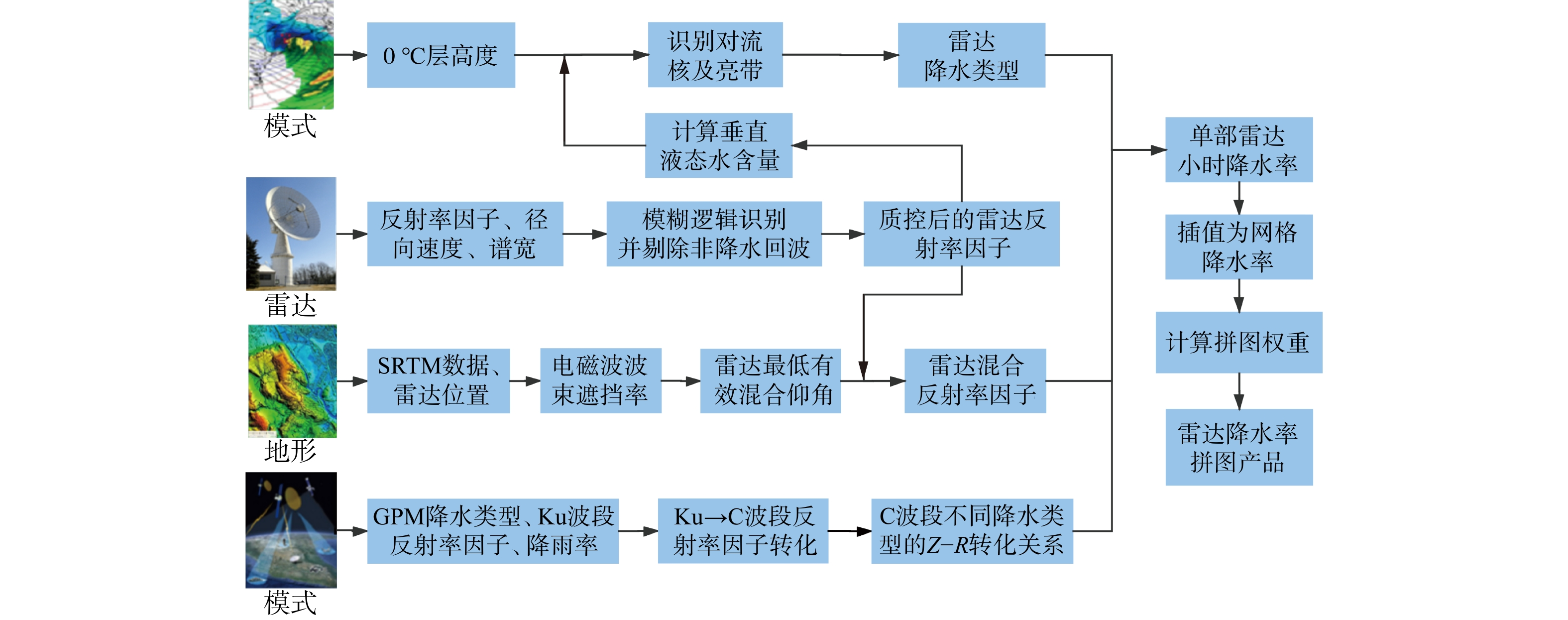图 2  雷达定量降水估计算法流程 Fig. 2  An overview flowchart of radar QPE algorithm
2.1 雷达探测资料质量控制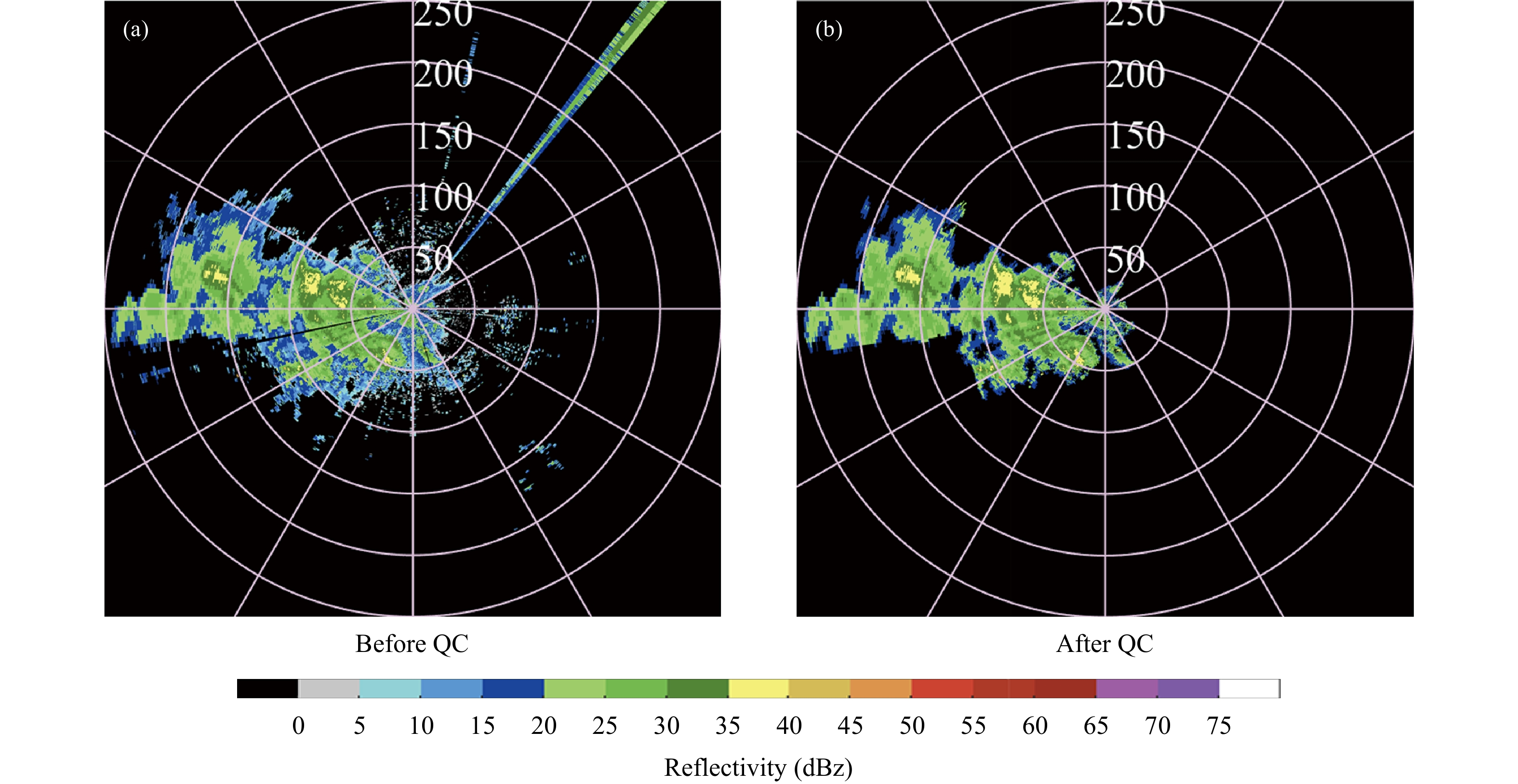图 3  2019年4月28日03时24分西安雷达0.5°仰角反射率回波 （a. 质量控制前，b. 质量控制后） Fig. 3  Reflectivity of Xi'an radar （a） before and （b） after QC at 0.5° elevation at 03：24 UTC 28 April 2019
2.2 雷达降水类型分类

2.2.1 0°C层高度检查

2.2.2 对流核识别

2.2.3 对流区识别

2.2.4 0℃层亮带区识别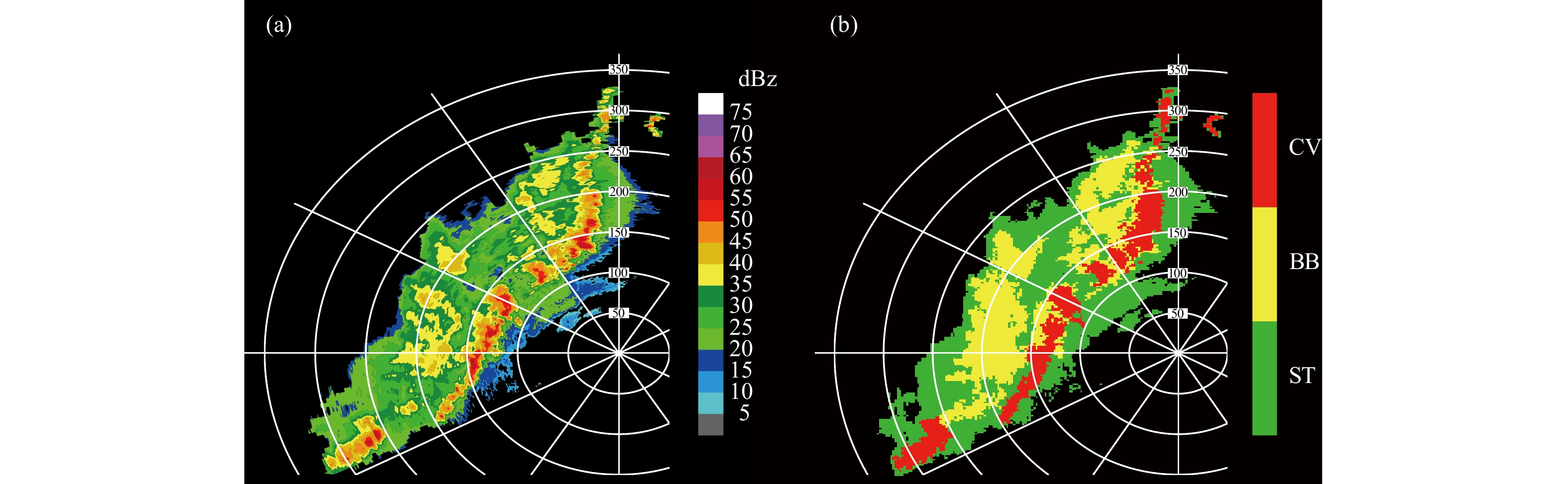图 4  （a） 雷达组合反射率和 （b） 降水类型分类结果 （CV、BB、ST分别代表对流性降水、0℃层亮带层状云降水和非0℃层亮带层状云降水） Fig. 4  （a） Composite reflectivity and （b） precipitation type （CV，BB，and ST stand for convective，bright band and stratiform，respectively）
2.3 雷达混合仰角反射率计算

 ${h_{\rm{c}}} = {\left({R_{\rm{e}}^2 + {r^2} + 2{R_{\rm{e}}}r\sin t} \right)^{1/2}} - {R_{\rm{e}}}$ (1)
 ${R_{\rm{e}}} = \frac{\,4\,}{\,3\,}{R_{\rm{0}}}$ (2)

 $d = 2r\sin \frac{\,\theta\, }{\,2\,}$ (3)

 $P = \int_0^d {\int_{{h_{\rm{b}}} - {h_{\rm{c}}}}^{y\left(x \right) - {h_{\rm{c}}}} {f\left({x,y} \right)} {\rm{d}}x{\rm{d}}y}$ (4)
 $f\left({x,y} \right) = \frac{1}{{2{{\text π }}{{\left| \boldsymbol\varSigma \right|}^{{1 / 2}}}}}\exp \left({ - \frac{1}{2\boldsymbol\varSigma}{{\left({{ X} - \overline { X} } \right)}^{\rm T}}\left({{ X} - \overline { X} } \right)} \right)$ (5)
 ${\boldsymbol X} = \left[ \begin{gathered} x \\ y \\ \end{gathered} \right],\quad\overline {\boldsymbol X} = \left[ \begin{gathered} \overline x \\ \overline y \\ \end{gathered} \right],\quad{{\boldsymbol\varSigma}} = \left[ {\begin{array}{*{20}{c}} {\sigma _{{x}}^2}&0 \\ 0&{\sigma _{{y}}^2} \end{array}} \right]$ (6)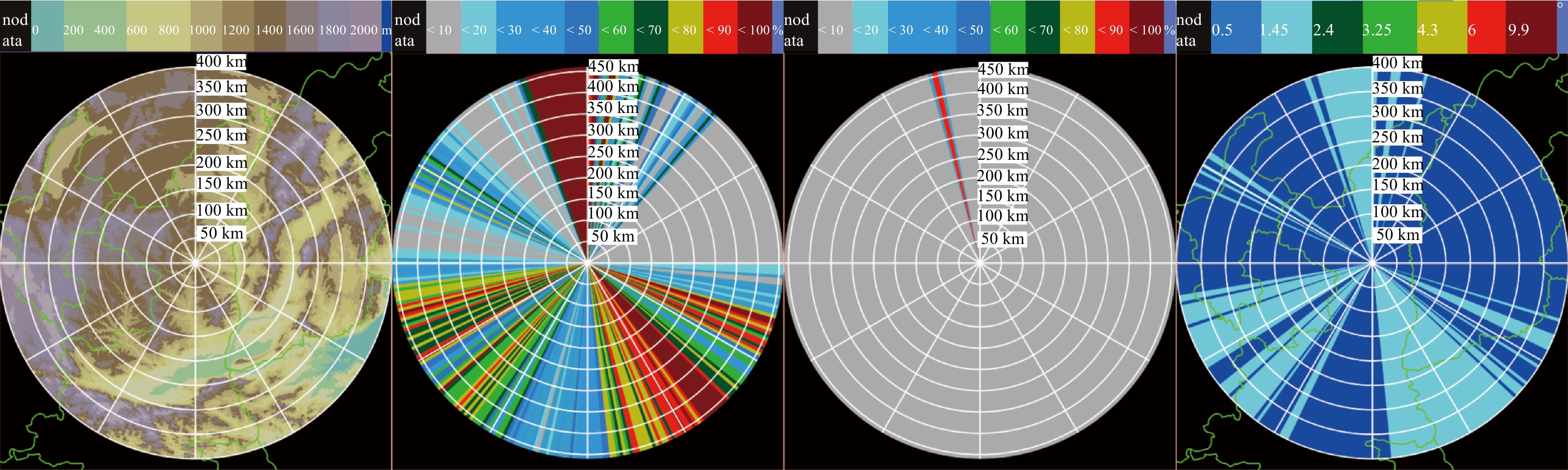图 5  延安雷达 （a） 扫描平面内地形空间分布及雷达（b） 0.5°、（c） 1.45°仰角电磁波遮挡百分比空间分布和 （d） 雷达混合仰角 Fig. 5  Distribution of （a） topography，electromagnetic wave shielding percentage at （b） 0.5° elevation and （c） 1.45° elevation，and （d） hybrid elevation angle of Yan'an radar
2.4 基于GPM星载双频雷达（DPR）观测统计分析Z-R关系

Zhu等（2020a2020b）利用全球降水测量任务（GPM）星载双频雷达（DPR）对陕西省降水时、空变化特征的分析得到了以下结论：（1）不同降水类型的垂直结构（微物理过程）具有明显的差异，（2）相同降水类型和强度的垂直反射率廓线在陕西省不同季节和不同地区的差异并不显著。因此，利用GPM卫星雷达反演的滴谱数据对不同降水类型的Z-R关系进行了统计分析，文中所用GPM卫星数据从地球数据网站（https://storm.pps.eosdis.nasa.gov/storm/）获取。

 $Z{\left(\lambda \right)_{\rm{h}}} = \frac{{4{\lambda ^4}}}{{{{{\text π }}^4}{{\left| {K_{\rm w}} \right|}^2}}}\int_0^{ + \infty } {{{\left| {{F_{{\rm{hh}}}}} \right|}^2}N\left(D \right){\rm{d}}D}$ (7)
 ${K_{\rm{w}}} = ({\varepsilon _{\rm{w}}} - 1)/({\varepsilon _{\rm{w}}} + 2)$ (8)
 $\begin{split}\int_0^{ + \infty } {{{\left| {{F_{{\rm{hh}}}}} \right|}^2}N\left(D \right){\rm{d}}D} =& \left({1 - 2{\sigma ^2}} \right)\int_0^{ + \infty } {{{\left| {{f_{\rm{a}}}} \right|}^2}N\left(D \right){\rm{d}}D} +\\& 2{\sigma ^2}\int_0^{ + \infty } {{f_{\rm{a}}}f_{\rm{b}}^ * N\left(D \right){\rm{d}}D} \end{split}$ (9)
 $N\left(D \right) = {N_{\rm{w}}}f\left(\mu \right){\left({\frac{D}{{{D_{\rm{0}}}}}} \right)^\mu }\exp \left({ - \varLambda D} \right)$ (10)
 $f\left(\mu \right) = \frac{6}{{{{3.67}^4}}}\frac{{{{\left({3.67 + \mu } \right)}^{\mu + 4}}}}{{\varGamma (\mu + 4)}},\quad\varLambda = \frac{{3.67 + \mu }}{{{D_{\rm{0}}}}}$ (11)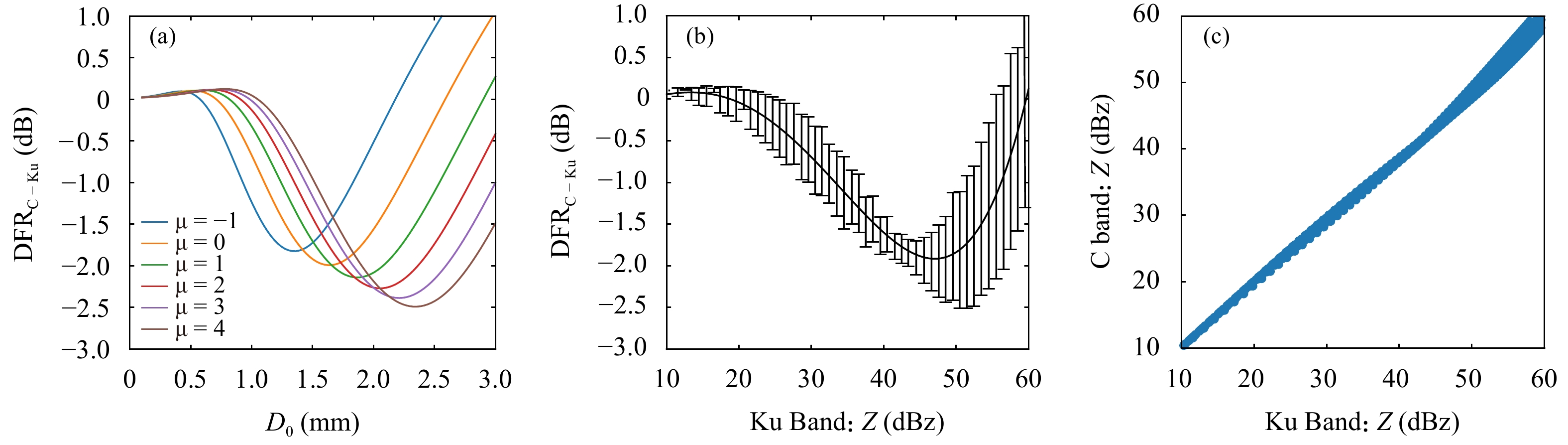图 6  Ku波段和C波段在雨区对降水粒子的反射特性 （a. $\mu$ 取−1—4时DFR随 ${D_0}$ 取值不同的变化；b. DFR随Ku波段降水粒子反射率不同产生的变化，黑色实线代表不同滴谱参数取平均后的结果，误差棒表示标准差；c. Ku波段和C波段反射率的散点分布） Fig. 6  Comparison of Ku-band and C-band reflection characteristics of precipitation particles in rainy areas （a） The change of DFR with the value of when μ is from −1 to 4；（b） the relationship of DFR with different reflectivity of precipitation particles in Ku-band，the black solid line represents the relationship with average of different drop spectrum parameters，error bars indicate standard deviation；and （c） scatter plot of reflectivity of Ku-band and C-band radar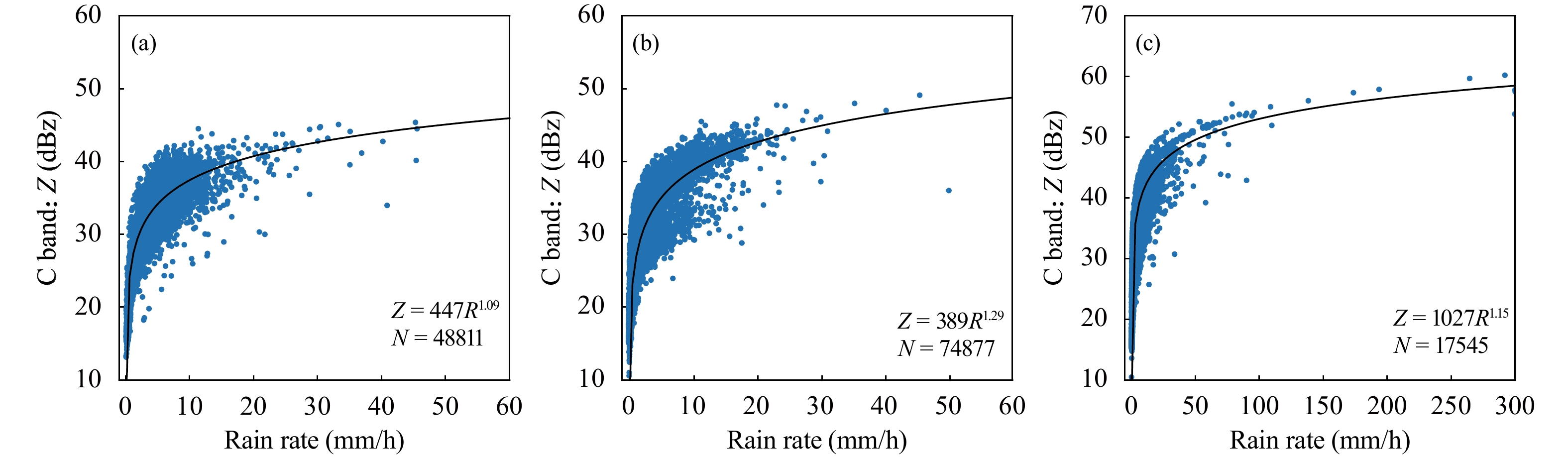图 7  不同降水类型中C波段雷达降水反演的Z-R关系 （适用于液态降水）（a. 0℃层亮带层状云降水，b. 非 0℃层亮带层状云降水，c. 对流性降水；黑色实线为拟合线） Fig. 7  The Z-R relationship of C-band radar precipitation for different precipitation types （applicable to liquid precipitation）（a. bright band，b. stratiform，and c. convective；The black solid line is the fitted line）
2.5 基于雷达观测原理的雷达拼图

 ${k_i} = \frac{{1000}}{{{h_{{\rm c},i}}}} + \frac{{1000}}{{{d_i}}}$ (12)
 ${w_i} = \frac{{{k_i}}}{{\displaystyle\sum\limits_{i = 1}^n {{k_i}} }}$ (13)图 8  2018年8月21日11时雷达拼图 （a. 雷达混合仰角反射率场，b. 降水类型分类场，c. 1 h雷达降水率场） Fig. 8  Multi-radar mosaic field of （a） reflectivity of hybrid elevation angle，（b） precipitation type，and （c） 1 h precipitation rate
3 结果分析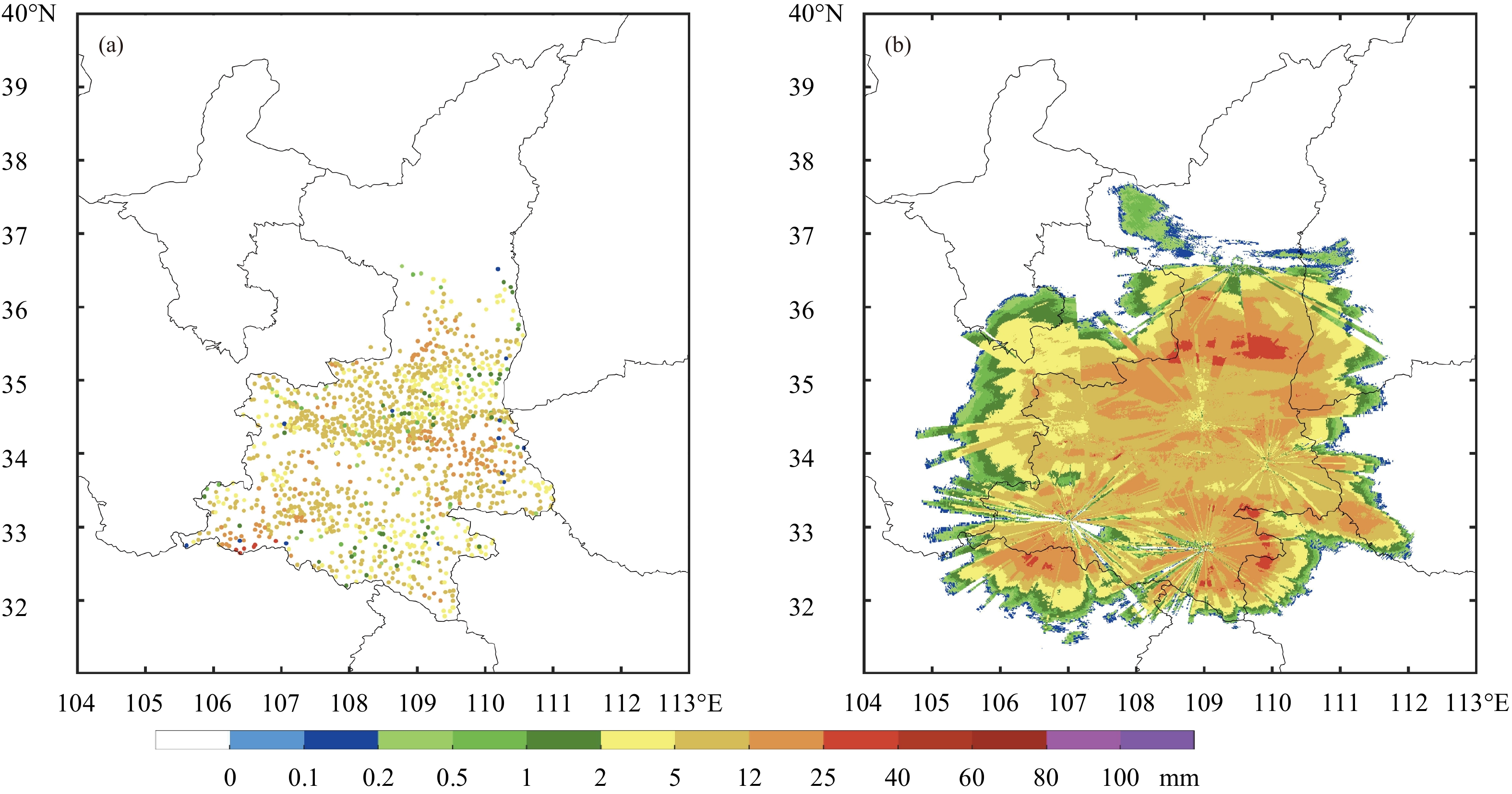图 9  2019年5月26日14时—29日02时累计降水空间分布 （a. 地面雨量站累计降水观测，b. 雷达定量降水估计算法计算得到的累计降水；单位：mm） Fig. 9  Accumulated precipitation for the period from 14：00 UTC 26 May to 02：00 UTC 29 May 2019 from （a） surface gauge stations and （b） QPE algorithm described in this paper （unit：mm）

（1）均方根误差（RMSE）

 ${\rm{RMSE}} = {\left[\frac{1}{N}\sum\limits_{k = 1}^N {{{({r_{\rm{k}}} - {g_{\rm{k}}})}^2}} \right]^{1/2}}$ (14)

（2）相对误差（RMAE）

 ${\rm{RMAE}} = \tfrac{{\displaystyle\sum\limits_{k = 1}^N {\left| {{r_{\rm{k}}} - {g_{\rm{k}}}} \right|} }}{{\displaystyle\sum\limits_{k = 1}^N {{g_{\rm{k}}}} }}$ (15)

（3）相对偏差（RMB）

 ${\rm{RMB}} = \tfrac{{\displaystyle\sum\limits_{k = 1}^N {\left({{r_{\rm{k}}} - {g_{\rm{k}}}} \right)} }}{{\displaystyle\sum\limits_{k = 1}^N {{g_{\rm{k}}}} }}$ (16)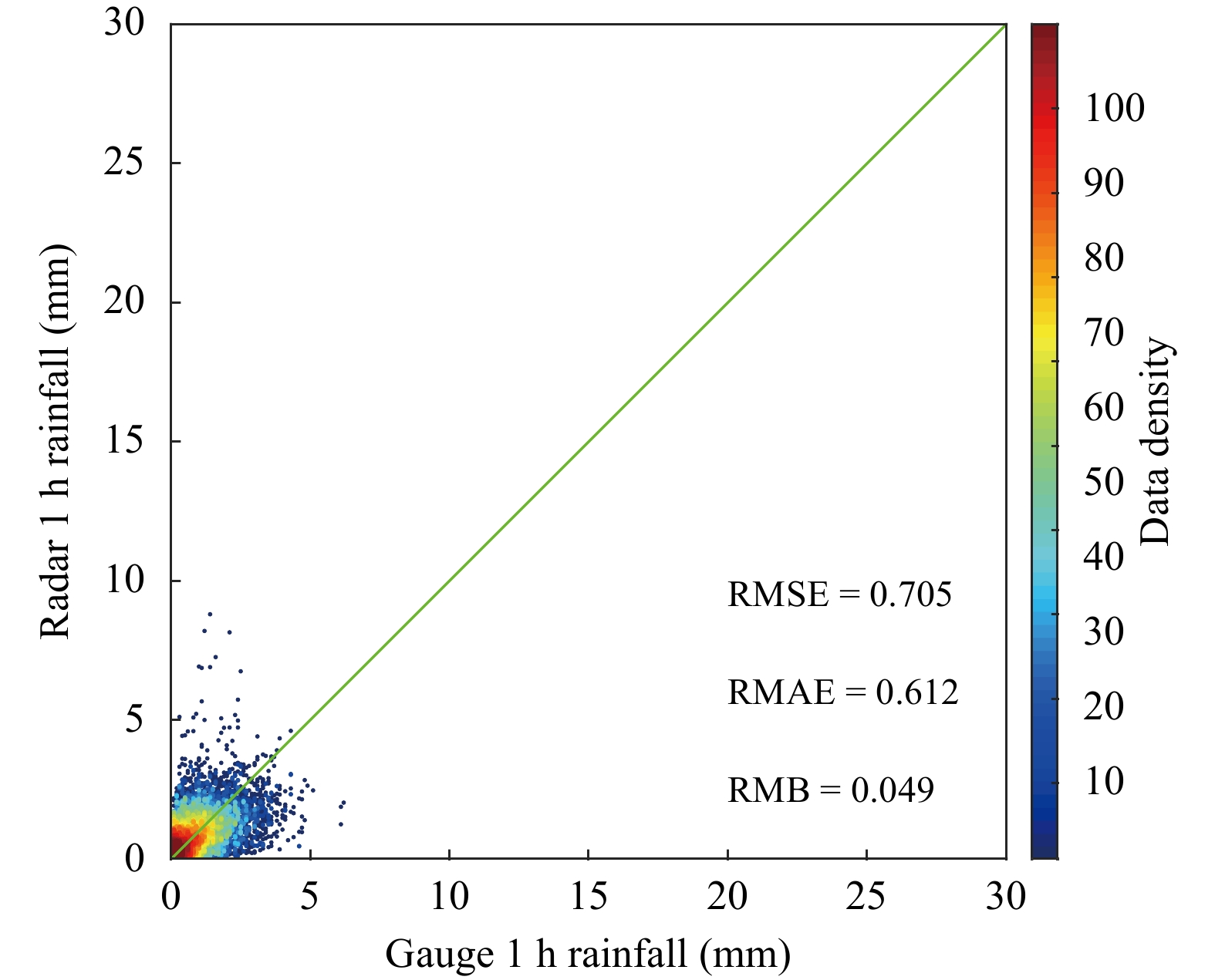图 10  2019年5月26日14时—29日02时1 h累计降水与地面雨量站观测降水散点对比 Fig. 10  Scatterplots of 1 h precipitation from QPE algorithm described in this paper vs the surface gauge station observations from 14：00 UTC 26 May to 02：00 UTC 29 May 2019

 个例 日期-时间 个例介绍 1 04-07 14:00—04-09 04:00 降水主要集中在陕西北部及南部地区，其中南部地区主要为对流性降水 2 04-26 01:00—04-28 09:00 飑线过程，自西北向东南移动，逐渐减弱成团状对流系统，降水主要集中在陕西中部及南部地区 3 05-05 15:00—05-08 22:00 降水主要集中在陕西中部及南部地区，其中中部地区有对流性降水过程 4 05-26 14:00—05-29 02:00 降水主要集中在陕西中部及南部地区，有对流性降水过程 5 06-26 14:00—06-28 14:00 全省范围内均有降水，其中南部地区主要为对流性降水 6 07-20 14:00—07-23 01:00 降水主要集中在陕西北部、中部和西南部地区，为对流性降水 7 07-28 14:00—07-31 08:00 全省范围内均有降水，其中北部和南部地区主要为对流性降水 总计 406 h 7次个例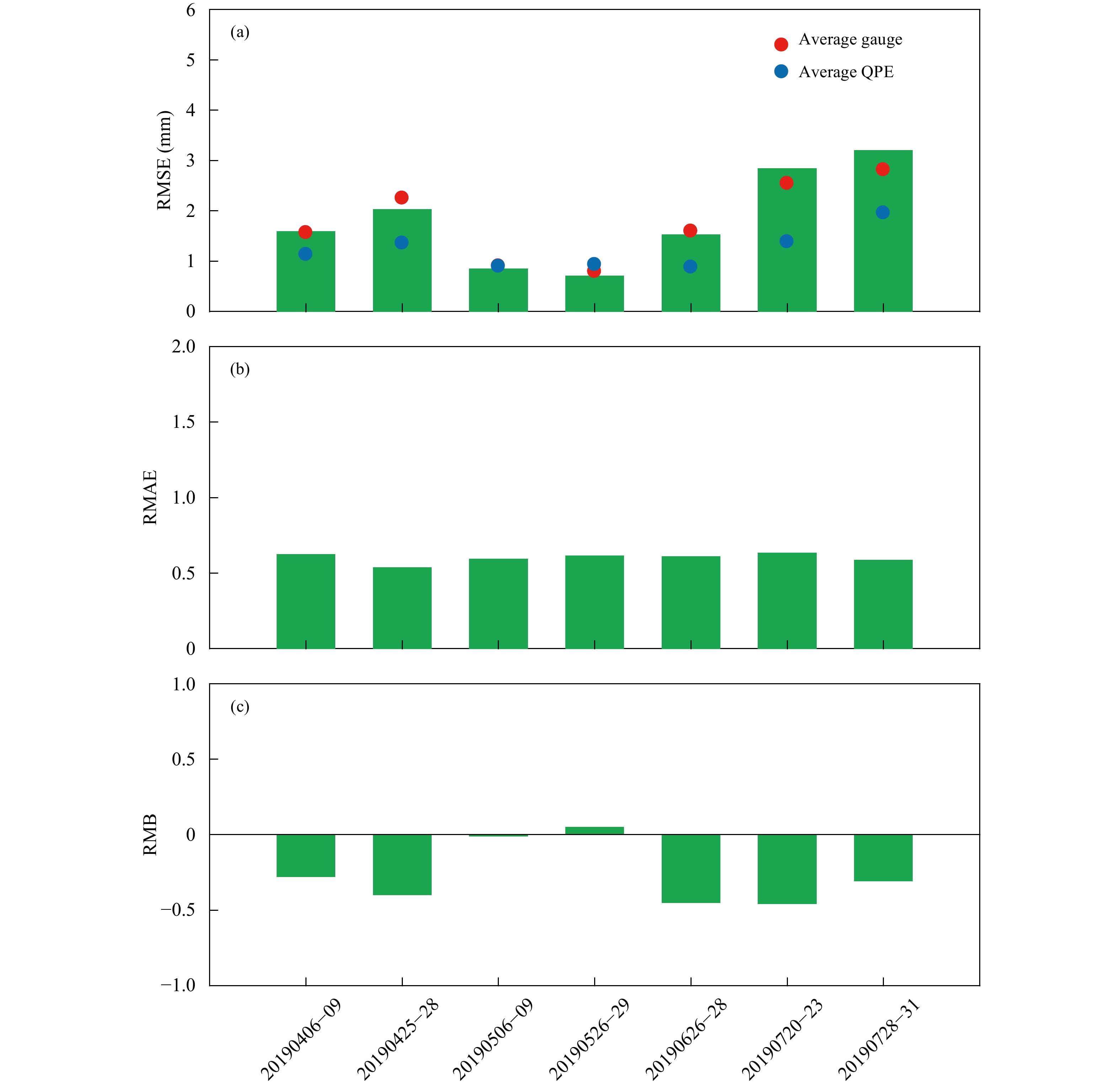图 11  针对陕西7次降水过程，文中定量降水估计算法结果和地面雨量站降水观测对比统计评分指标 （a. 均方根误差，b. 相对误差，c. 相对偏差） Fig. 11  Statistical indicators of （a） RMSE，（b） RMAE，and （c） RMB for 7 precipitation processes in Shaanxi

4 结论与讨论

（1）雷达探测资料质量控制算法对地物回波、尖峰等非降水回波和降水回波识别效果较好，能够较好地保留降水信息，但对于降水系统边缘较弱的气象回波有误识别现象，算法需要进一步改进。

（2）通过雷达降水类型分类算法，能够较准确地识别对流性降水、非0℃层亮带层状云降水和0℃层亮带层状云降水。

（3）根据陕西地区雷达遮挡情况，设计了混合最低有效仰角反射率算法，可有效降低地形遮挡导致的降水低估或缺失现象。

（4）利用GPM卫星雷达反演的滴谱数据对不同类型降水的Z-R关系进行了统计分析，针对不同降水类型采用对应的Z-R关系有利于提高QPE精度。

（5）文中选取了7次降水过程，对新算法生成的雷达QPE产品进行了评估，雷达算法相对稳定，相对误差、相对偏差指标也均表明该算法结果与地面雨量站降水观测结果接近。